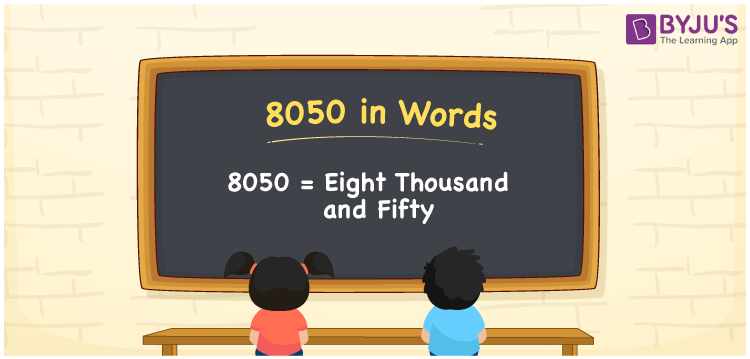# 8050 in Words

8050 in words can be written as Eight Thousand and Fifty. If you buy a refrigerator for Rs. 8050, then you can say that “I bought a refrigerator for Eight Thousand and Fifty Rupees”. You will learn clearly about how numbers in words can be written using the English alphabet. Therefore, 8050 can be read as “Eight Thousand and Fifty” in words.

 8050 in words Eight Thousand and Fifty Eight Thousand and Fifty in Numbers 8050

## 8050 in English Words## How to Write 8050 in Words?

Students will learn how to write the place value chart of the number 8050 from the explanation provided below. Also you will learn the place value of each digit as it is designed by the experts according to the intelligence quotient of students.

 Thousands Hundreds Tens Ones 8 0 5 0

8050 in expanded form is:

8 x Thousand + 0 × Hundred + 5 × Ten + 0 × One

= 8 x 1000 + 0 × 100 + 5 × 10 + 0 × 1

= 8000 + 50

= 8050

= Eight Thousand and Fifty

Therefore, 8050 in words is written as Eight Thousand and Fifty.

8050 is a natural number that precedes 8051 and succeeds 8049.

8050 in words – Eight Thousand and Fifty

Is 8050 an odd number? – No

Is 8050 an even number? – Yes

Is 8050 a perfect square number? – No

Is 8050 a perfect cube number? – No

Is 8050 a prime number? – No

Is 8050 a composite number? – Yes

## Frequently Asked Questions on 8050 in Words

Q1

### Write 8050 in words.

8050 can be written in words as “Eight Thousand and Fifty”.
Q2

### How do you write Eight Thousand and Fifty in numbers?

Eight Thousand and Fifty can be written in numbers as 8050.
Q3

### Is 8050 an even number?

Yes, 8050 is an even number as it is completely divisible by 2.